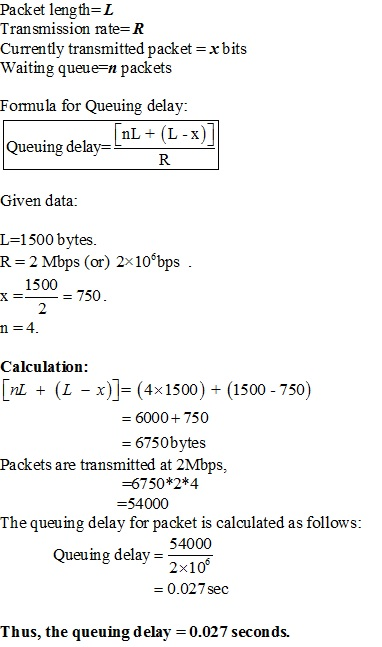SHARE
HELP

To start with, Sr2Jr’s first step is to reduce the expenses related to education. To achieve this goal Sr2Jr organized the textbook’s question and answers. Sr2Jr is community based and need your support to fill the question and answers. The question and answers posted will be available free of cost to all.Authors:
James F. Kurose, Keith W. Ross
Chapter:
Computer Networks And The Internet
Exercise:
Problems
Question:12 | ISBN:9780132856201 | Edition: 6

###Question

A packet switch receives a packet and determines the outbound link to which

the packet should be forwarded. When the packet arrives, one other packet is halfway done being transmitted on this outbound link and four other packets are waiting to be transmitted. Packets are transmitted in order of arrival.

Suppose all packets are 1,500 bytes and the link rate is 2 Mbps. What is the queuing delay for the packet? More generally, what is the queuing delay when all packets have length L, the transmission rate is R, x bits of the currently-being-transmitted packet have been transmitted, and n packets are already in the queue?

###Answer

Consider the given data:###Geroge

Given data:
L=Length of packet=1500 bytes
R=Rate of transmission =2Mbps
x=Current transmit packet=L/R=1500/2=750
n=Waiting queue=4
Queuing delay=(nL+(L-x))/R
=(4*1500)(1500-750)/2
=6750/2
=3375 Mbps
=0.033 seconds

###Anon

The value 0.027 seconds is correct but the method is unclear. The other comment provides the correct formula but mixes up units.

L (packet length) = 1500 bytes

R (transmission rate) = 2 Mbps

x (currently transmitting packet) = L / 2 = 750 bytes

n (number in queue) = 4 packets

Queuing Delay = (nL + (L – x)) / R

(nL + (L – x)) / R = (4 * 1500 + (1500 – 750)) bytes / 2 Mbps

= 6570 bytes / 2 Mbps

= 0.027 Mb / 1 Mbps

= 0.027 seconds

Post the discussion to improve the above solution.